Total: 1117#### Animation tutorial#### Apps tutorial#### Desktop icons tutorial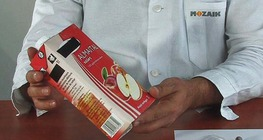#### The volume and surface area of a juice box

In this video we calculate the volume and surface area of a rectangular cuboid: a juice box.#### Elastic deflection

After the impact, the basketball regains its original shape, that is, elastic deflection occurs.#### Xylophone tutorial#### Wildlife tutorial#### Toy clock tutorial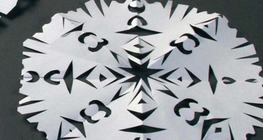#### Snowflake

Fold the paper here and there, chip one or two with the scissors. This is all you need to create something fantastic that looks like a snowflake.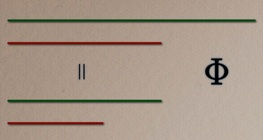#### Calculating the golden ratio

This video presents how to calculate the golden ratio.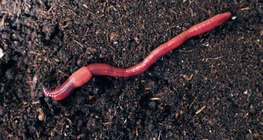#### Earthworm

You can always find earthworms in humid soil with sufficient humus content.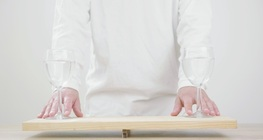#### Archimedes' seesaw

In this experiment, we use buoyancy to study the balance of two glasses of water.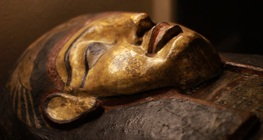#### Sarcophagus

The recording shows a more than 2000-year-old gilded sarcophagus. You can see the names of the deceased and his protecting gods on the coffin.#### 35mm cinema projector

Watch this video to learn how a cinema projector works and how a film appears on the screen.#### Reflecting a circle across an axis

Axis t and a circle with centre O and radius r are given on a particular plane. Let’s plot the mirror image of the circle.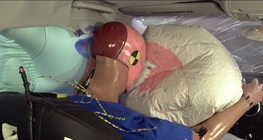#### How airbags work

A compound called sodium azide is responsible for the operation of airbags.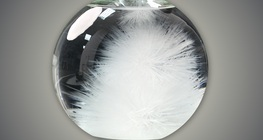#### The hot ice experiment

The words hot and ice together? There must be a trick somewhere. Let’s have a look.#### Plotting a perpendicular to a line at a given point P on the line

Both line e and point P, which is situated on it, are given. Let’s plot line g to include point P on line e so that it is perpendicular to line e.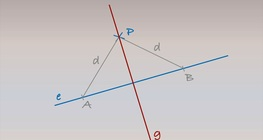#### Plotting a perpendicular on a line from a point P that is not on the line

Both line e and point P, which is not situated on line e, are given. Let’s plot line g such that it passes through point P and is perpendicular to line e.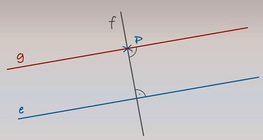#### Plotting parallel lines – Solution I

Let’s plot line g such that it is parallel to line e and passes through point P.